3.7 Modular Arithmetic

3.7.1 Introduction:

 Let us look at the Calendar of May 2006 which is given on the right hand side. 1st date of the month is Monday. What day is 29th of that month? It is Monday again. How did we arrive at that? If 1st is Monday then next Mondays are 1,8,15,22,29 Why  is this  so? This is because days repeat after every 7 days. Mathematically we say 8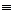1(modulo 7) which implies that 7 exactly divides (8-1) 151(mod 7) which implies that 7 exactly divides (15-1) 221(mod 7) which implies that 7 exactly divides (22-1)   In general when xy(mod m ) we mean  that m exactly divides (x-y). The above statement can also be represented by If x-y0(mod m) then (x-y)/m  = is an integer. ‘’ is pronounced as congruent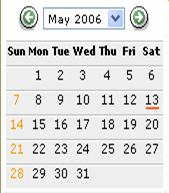We know that when we divide any positive integer by another positive integer say ‘m’, the reminders has to be among the set {0,1,2,3,4…..(m-1)}.

The numbers 0,1,2,….(m-1) are called residues of mod m.

The set Zm= {0,1,2,3,4…..(m-1)}is called the set of mod m.

Definition :  The ‘residue set’ of mod m is  the possible set of reminders when a number is divided by m and is represented by

Zm= {0,1,2,3,4…..(m-1)}

3.7.1 Example 1:  Write the residues when a positive integer is divided by 10

Solution :

The possible reminders when 10 is a divisor are  0,1,2,3….9

Therefore Z10= {0,1,2,3,4,5,6,7,8,9}

Will it be possible to define addition and multiplication operations under the modulo system?

Definition:   If mod is m then

The symbol for addition is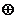with m as subscript to the symbol.

The symbol for multiplication is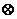with m as subscript to the symbol.

We define

1.  a +m br (=reminder of (a+b)/m)

2.  am br (=reminder of (a*b)/m)

3.7.1 Example 2 : Find 10 +12 2 +12 3

Solution :

10 +12 2 +12 3

=(10 +12 2) +12 3

=0+12 3(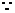reminder of (10+2)/12 = 0)

= 3(reminder of 3/12 is 3)

3.7.1 Example 3 : Find 411 311 7

Solution :

411 311 7

=(411 3)11 7

= 111 7(reminder of (4*3)/11 = 1)

=7 (reminder of (1*7)/11 = 7)

3.7.1 Example 4 : What is the value of y if  yy1(mod 8)

Solution :

Since yy1(mod 8)

8 should divide y*y with reminder = 1(y2-1)/8 = 0.

This is possible only if y=3.

Verification:

38 3 =1(reminder of (3*3)/8 = 1) which is as given in the problem.

3.7.2 Caley’s Table:

The Caley’s table is a representation of modular arithmetic operation.

The results of modulo operations (addition and multiplication) on a given set, when represented in a table format is called Caley’s table.

Let us represent Caley’s table for addition operation (ab) for mod 4.

Since the residues of mod 4 can only be 0 or 1 or 2 or 3, we calculate a+4b only for a =0,1,2,3

and b= 0,1,2,3.The set on which Caley’s table is arrived at is Z4= {0,1,2,3}

The results of  the operation [ab mod 4]

(denoted by +4)

0 +4 00           0 +4 11           0 +4 22      0 +4 33

1 +4 01           1 +4 12           1 +4 23      1 +4 30

2 +4 02           2 +4 13           2 +4 20      2 +4 31

3 +4 03           3 +4 10           3 +4 21      3 +4 32

 bà 0 1 2 3 a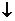a +4 b = 0 0 1 2 3 1 1 2 3 0 2 2 3 0 1 3 3 0 1 2

Observe  that the results in the table (Green Color Numbers) also belong to the set Z4= {0,1,2,3}

Since the residues of mod 4 can only be 0 or 1 or 2 or 3, we calculate a X4b  only for a =0,1,2,3

and b= 0,1,2,3.The set on which Caley’s table is arrived at is Z4= {0,1,2,3}

The results of  the operation [ab mod4] (denoted by X4)

0 X4 00           0 X4 10           0 X4 20      0 X4 30

1 X4 00           1 X4 11           1 X4 22      1 X4 33

2 X4 00           2 X4 12           2 X4 20      2 X4 32

3 X4 00           3 X4 13           3 X4 22      3 X4 31

 bà 0 1 2 3 aa4 b = 0 0 0 0 0 1 0 1 2 3 2 0 2 0 2 3 0 3 2 1

Observe  that the results in the table (Green Color Numbers) also belong to the set Z4= {0,1,2,3}

3.7.2 Problem  1 :  Construct Caley’s Table for Q ={0,2,4,6,8} undermod 10

Solution :

We are required to arrive at values of ab mod 10 when a, b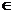to the set Q= {0,2,4,6,8}

Since 0 is reminder of (6+4)/10, 2 is reminder of (6+6)/10   and 4 is reminder of (8+6)/10

We get

6 +10 40           6 +10 62           8 +10 64

Similarly we can arrive at the remainders for other values of a and b.

 bà 0 2 4 6 8 a a +10 b= 0 0 2 4 6 8 2 2 4 6 8 0 4 4 6 8 0 2 6 6 8 0 2 4 8 8 0 2 4 6

3.7.2 Problem  2 :  Construct Caley’s Table for A = {1,5,7,11} undermod 12

We are required to arrive at values of ab mod 12 when a, bto the set A ={1,5,7,11}

Since 1 is reminder of (7*7)/12 , 5 is reminder of (7*11)/12 and 1 is reminder of (11*11)/12

We get

71271        712 115           1112 111

Similarly we can arrive at the remainders for other values of a and b.

 bà 1 5 7 11 a a12 b = 1 1 5 7 11 5 5 1 11 7 7 7 11 1 5 11 11 7 5 1

3.7 Summary of learning

 No. Points  learnt 1 Definition of Modulo operations 2 Modulo addition and multiplication 3 Caley’s Table